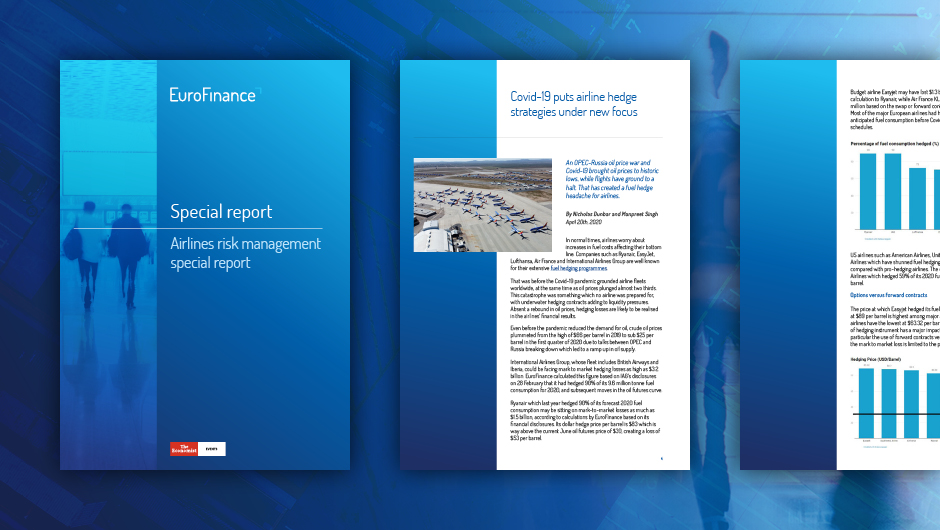Array (  => 1  => 6  => 4  => 4  => 5  => 0  => 1  => 4  => 1  => 7  => 1  => 3  => 2  => 3  => 4  => 3  => 1  => 2  => 2  => 2  => 5  => 1  => 3  => 1  => 4  => 0  => 3  => 0  => 1  => 2  => 2  => 2  => 2  => 1  => 4  => 6  => 1  => 3  => 5  => 5  => 2  => 2  => 4  => 3  => 2  => 2  => 2  => 0  => 1  => 1  => 4  => 3  => 4  => 2  => 3  => 0  => 2  => 3  => 2  => 3  => 2  => 0  => 2  => 2  => 0  => 3  => 2  => 3  => 2  => 1  => 3  => 4  => 1  => 5  => 3  => 4  => 3  => 2  => 5  => 2  => 3  => 2  => 2  => 2  => 1  => 3  => 0  => 2  => 3  => 0 )
Browse topics and content
• airlines
• commodity risk
• Hedging

## Special report: Airlines risk managementAirline hedging travels new route since Covid-19

All treasurers whose companies consume raw materials to provide services or products must think about commodity price risk. But few have seen challenges such as airlines have faced since the start of the Covid-19 pandemic.

Flights were cancelled as countries shut their borders and instituted lockdowns. The result was billions of losses, and an eventual shakeup of airline risk management practices.

EuroFinance has covered these developments step by step. It was our independent reporting that first identified a problem with fuel hedging ineffectiveness in April 2020, and predicted the eventual losses. The prediction would be vindicated in the following months, as documented in further articles.

Download the Airlines Risk Management special report to follow this journey from the start of the pandemic to the present day as airlines are learning lessons and thinking about things in a new way.